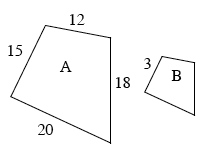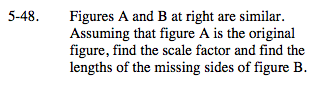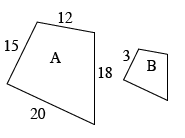### Home > MC2 > Chapter 5 > Lesson 5.2.1 > Problem5-48

5-48.

Figures A and B below are similar. Assuming that figure A is the original figure, find the scale factor and find the lengths of the missing sides of figure B. Homework Help ✎See problem 4-98 for help with scale factor and solving for missing lengths.

Which side of the larger quadrilateral corresponds to the side length of 3 on the smaller quadrilateral?
What is the ratio between the corresponding side lengths?

$\text{New sides: 4, } \frac{18}{5} \text{, and } \frac{12}{5}$

What would the scale factor be?